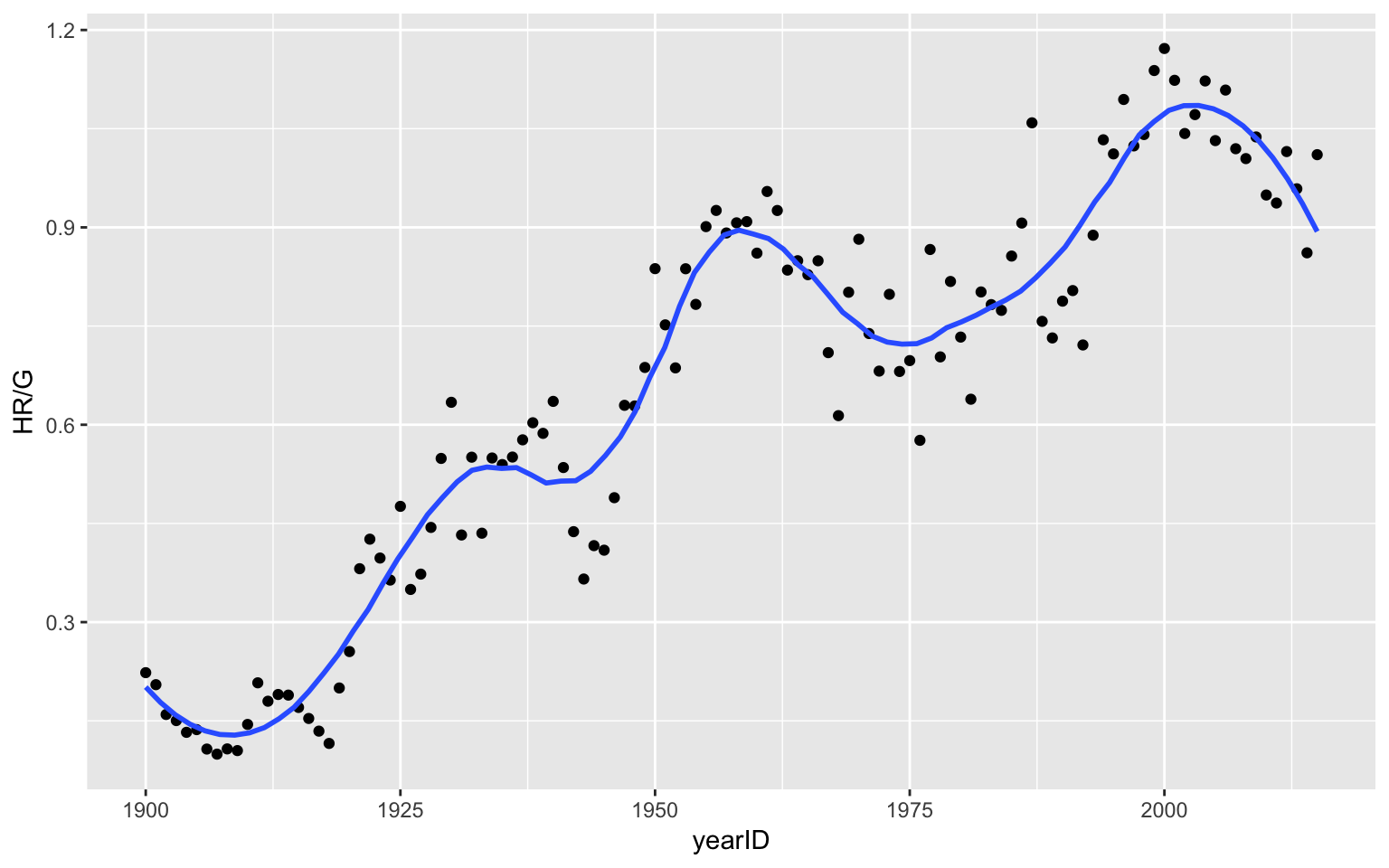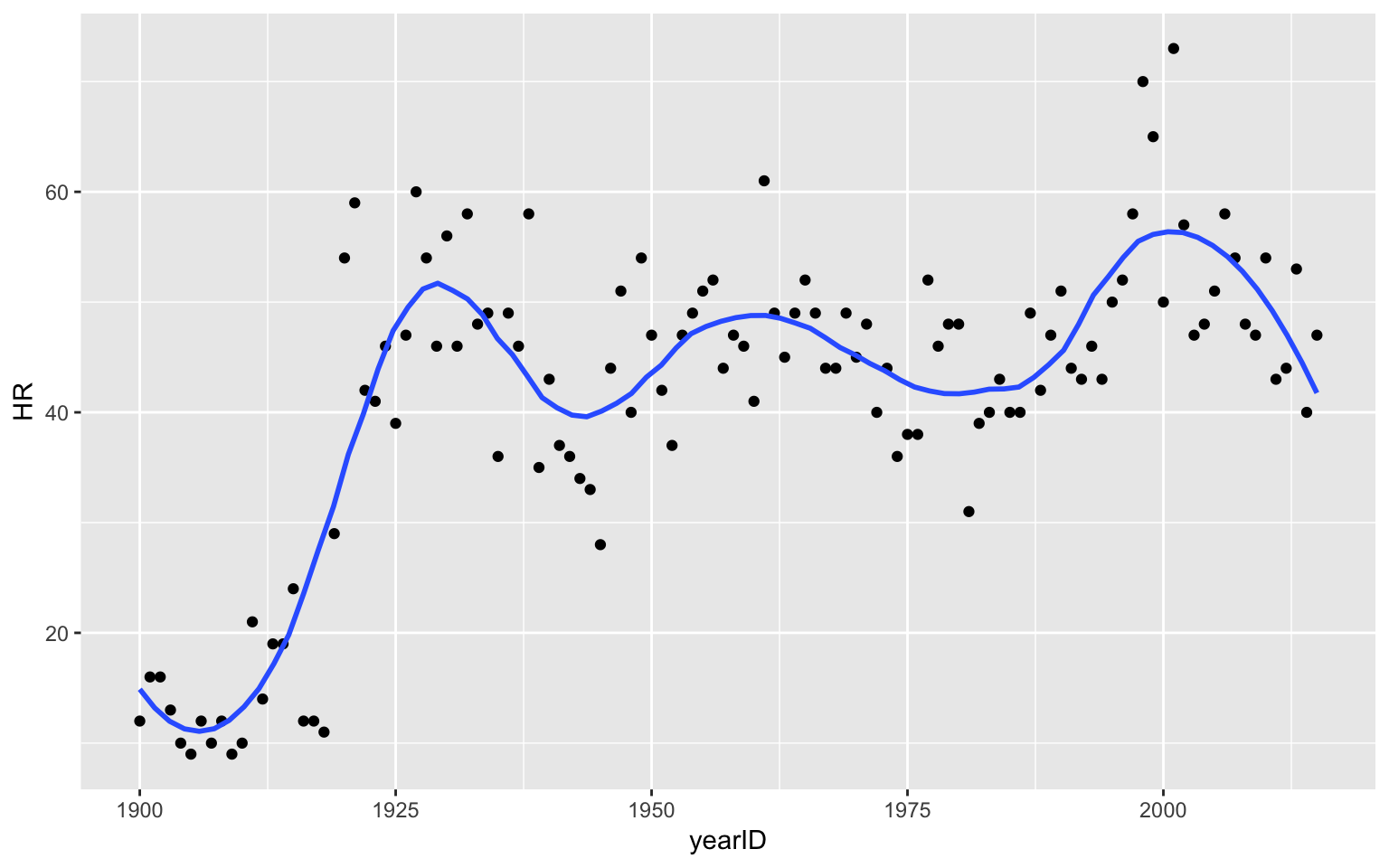### History of Home Run Hitting

We load the packages Lahman, dplyr and ggplot2.

library(Lahman)
library(dplyr)
library(ggplot2)

#### The Data

The Lahman package contains season to season data for players and teams from the Sean Lahman database. For this history of home runs graph, want to collect the number of home runs hit (variable HR) and number of games played (variable G) for all teams for all seasons since 1900.

Here are a few sample rows of our data.

select(sample_n(Teams, 8), yearID, teamID, HR, G)
##      yearID teamID  HR   G
## 2625   2009    WAS 156 162
## 1664   1975    BOS 134 160
## 2252   1997    MIN 132 162
## 491    1907    PHI  12 149
## 2291   1998    SFN 161 163
## 1180   1949    PIT 126 154
## 1924   1985    CIN 114 162
## 1895   1984    CAL 150 162

#### Creating the Variables of Interest

I use the summarize function to collect the total number of home runs hit and the total number of games played for each season.

S <- summarize(group_by(Teams, yearID),
HR = sum(HR),
G = sum(G))

I use the filter function to select the summary data for only seasons 1900 or later.

S2 <- filter(S, yearID >= 1900)

#### Constructing the Graph

Now I can construct the plot. I construct a scatterplot of yearID (horizontal) against the number of home runs hit for each team per game (variable HR / G). I add a smoothing loess curve to the plot to see the general pattern.

ggplot(S2, aes(yearID, HR / G)) +
geom_point() +
geom_smooth(method="loess", span=0.3, se=FALSE)### History of Home Run Leaders

Next suppose we want to graph the leading number of home runs against season for all seasons past 1900.

#### The Data

The Batting data frame in the Lahman package contains the number of home runs hit by each player each season.

#### Creating the Variables of Interest

Actually, the Batting table contains more than one row for players who play for more than one team in a given season. So the first use the summarize function to find the number of home runs for each player each season.

S <- summarize(group_by(Batting, yearID, playerID),
HR=sum(HR, na.rm=TRUE))

Next, for each season, we collect the maximum number of home runs that are hit. Also we select only the rows of the data frame where the season is at least 1900.

S1 <- summarize(group_by(S, yearID),
HR=max(HR, na.rm=TRUE))
S2 <- filter(S1, yearID >= 1900)

Here are the first few rows of our table.

head(S2)
## # A tibble: 6 x 2
##   yearID    HR
##    <int> <int>
## 1   1900    12
## 2   1901    16
## 3   1902    16
## 4   1903    13
## 5   1904    10
## 6   1905     9

#### Constructing the Graph

Now I can construct the plot. I construct a scatterplot of yearID (horizontal) against the maximum number of home runs hit (variable HR). I add a smoothing loess curve to the plot to see the general pattern.

ggplot(S2, aes(yearID, HR)) +
geom_point() +
geom_smooth(method="loess", span=0.3, se=FALSE)